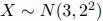# Statistical Computation and Simulation

Many of the tedious statistical computations that would once have had to have been done from statistical tables can be easily carried out in R. This can be useful for finding confidence intervals etc. Let’s take as an example the Normal distribution. There are functions in R to evaluate the density function, the distribution function and the quantile function (the inverse distribution function). These functions are, respectively, dnorm, pnorm and qnorm. Unlike with tables, there is no need to standardize the variables first. For example, suppose, then > dnorm(x,3,2) will calculate the density function at points contained in the vector x (note, dnorm will assume mean 0 and standard deviation 1 unless these are specified. Note also that the function assumes you will give the standard deviation rather than the variance. As an example

> dnorm(5,3,2)

 0.1209854

evaluates the density of the N(3;4) distribution at x= 5. As a further example

> x<-seq(-5,10,by=.1)

> dnorm(x,3,2)

calculates the density function of the same distribution at intervals of 0.1 over the range [¡5;10].

The functions pnorm and qnorm work in an identical way.

Similar functions exist for other distributions. For example, dt, pt and qt for the t-distribution, though in this case it is necessary to give the degrees of freedom rather than the mean and standard deviation. Other distributions available include the binomial, exponential, Poisson and gamma, though care is needed interpreting the functions for discrete variables.

One further important technique for many statistical applications is the simulation of data from specified probability distributions. R enables simulation from a wide range of distributions, using a syntax similar to the above. For example, to simulate 100 observations from the N(3;4) distribution we write

> rnorm(100,3,2)

Similarly, rt, rpois for simulation from the t and Poisson distributions, etc.